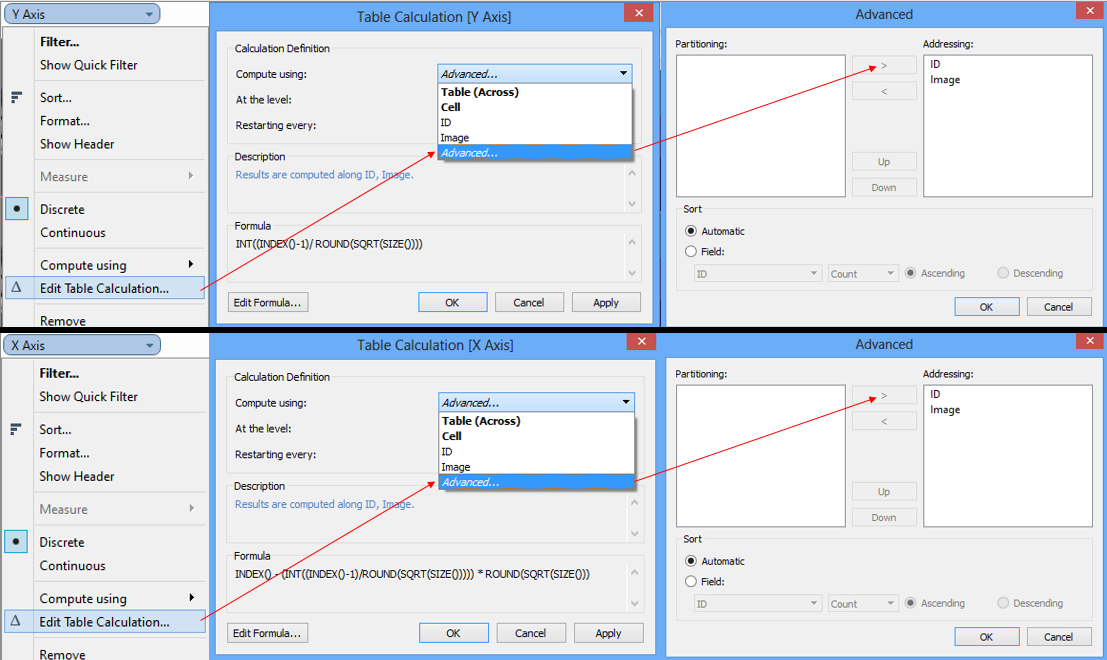## Sunday, September 28, 2014

### How to auto-scale and auto-arrange shapes/visuals in Tableau

I ran into a unique problem when I built my sports viz a while back.  I had this idea to show a picture for every value of a metric.  However, when I sized the sheet to fit the maximum allowable values, any less than the maximum showed a large amount of white space. (I've since updated the sports viz with this article's trick)

Then my friend Steven Carter released his viz titled "The Adoption Gap" (and subsequently won Viz of the Day) where he took my picture idea to the next level with his household grid.  Using formulas, he was able to set a grid, and then show a gradually filled in house to represent the number of adoptions needed.

AND THEN Chris Luv released his viz explaining INDEX() and SIZE(), two incredibly valuable table calculations in tableau to determine size and position of a point.  Using INDEX and SIZE, he was able to completely recalculate row and column values on the fly, removing a lot of constraints Steven and I had come up with. His final viz was so good, it made me rethink my entire design.

Having been inspired by a number of sources, I present a fourth option (very close to Chris' solution but with a slight tweak). I have build a sample viz that shows how to use formulas and table calculations to autoscale and autoalign shapes (or visualizations as Chris will show) to a grid based on how many values are available. Check out the final result below.

### HOW TO

In my original sports viz, I was unaware that Tableau will do the sizing and arranging work for you if you have rows and columns that the images will fit in.  So here's how to use a unique ID column to auto generate the links we need

1) Create 'Y Axis' Field

INT((INDEX()-1)/ ROUND(SQRT(SIZE())))

So what is going on here?  The idea is that as the number of objects to display is usually best represented in equal rows and columns.  Thus, the number of rows we want (represented on the Y axis) should be the square root of the objects/dimension values.  The INDEX() -1 offsets the values so they continue to line up instead of shifting out.

2)  Create 'X Axis' Field

INDEX() - (INT((INDEX()-1)/ROUND(SQRT(SIZE())))) * ROUND(SQRT(SIZE()))

This one is a bit more complicated, but is to continue to hit on the idea of displaying equal number of rows and columns.  So with the 'Y Axis' figuring out a bucket for values for the row, the X axis needs to reassign the ID for the value so that the rows start over successfully.

So what this formula is doing is taking the current index value, subtracting the y axis bucket multiplied by the bucket value to always reestablish the new set of rows.

3) Build sheet and assign shapes

The setup is as follows
a) [ID] goes in the details
b) 'X Axis' goes on the columns shelf, convert to discrete
c) 'Y Axis' goes on the rows shelf, convert to discrete
d) 'Y Axis' and 'X Axis' should be addressing every field (see second screenshot for the how to)a), b), & c)d)
Let me know if this is helpful or if you have any questions.  Thank you!